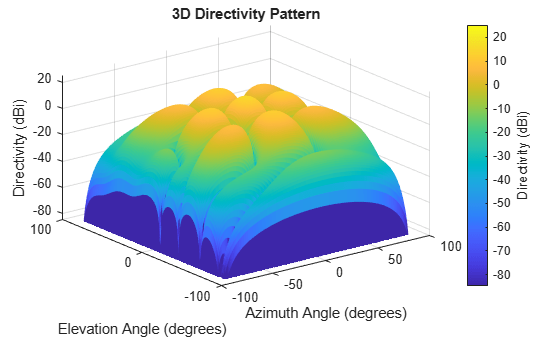# step

System object: phased.HeterogeneousURA
Package: phased

Output responses of array elements

## Syntax

```RESP = step(H,FREQ,ANG) ```

## Description

Note

Starting in R2016b, instead of using the `step` method to perform the operation defined by the System object™, you can call the object with arguments, as if it were a function. For example, `y = step(obj,x)` and `y = obj(x)` perform equivalent operations.

`RESP = step(H,FREQ,ANG)` returns the array elements’ responses `RESP` at operating frequencies specified in `FREQ` and directions specified in `ANG`.

Note

The object performs an initialization the first time the object is executed. This initialization locks nontunable properties and input specifications, such as dimensions, complexity, and data type of the input data. If you change a nontunable property or an input specification, the System object issues an error. To change nontunable properties or inputs, you must first call the `release` method to unlock the object.

## Input Arguments

 `H` Array object `FREQ` Operating frequencies of array in hertz. `FREQ` is a row vector of length L. Typical values are within the range specified by a property of `H.Element`. That property is named `FrequencyRange` or `FrequencyVector`, depending on the type of element in the array. The element has zero response at frequencies outside that range. `ANG` Directions in degrees. `ANG` is either a 2-by-M matrix or a row vector of length M. If `ANG` is a 2-by-M matrix, each column of the matrix specifies the direction in the form ```[azimuth; elevation]```. The azimuth angle must lie between –180° and 180°, inclusive. The elevation angle must lie between –90° and 90°, inclusive. If `ANG` is a row vector of length M, each element specifies the azimuth angle of the direction. In this case, the corresponding elevation angle is assumed to be 0°.

## Output Arguments

 `RESP` Voltage responses of the phased array. The output depends on whether the array supports polarization or not. If the array is not capable of supporting polarization, the voltage response, `RESP`, has the dimensions N-by-M-by-L. N is the number of elements in the array. The dimension M is the number of angles specified in `ANG`. L is the number of frequencies specified in `FREQ`. For any element, the columns of `RESP` contain the responses of the array elements for the corresponding direction specified in `ANG`. Each of the L pages of `RESP` contains the responses of the array elements for the corresponding frequency specified in `FREQ`.If the array is capable of supporting polarization, the voltage response, `RESP`, is a MATLAB `struct` containing two fields, `RESP.H` and `RESP.V`. The field, `RESP.H`, represents the array’s horizontal polarization response, while `RESP.V` represents the array’s vertical polarization response. Each field has the dimensions N-by-M-by-L. N is the number of elements in the array, and M is the number of angles specified in `ANG`. L is the number of frequencies specified in `FREQ`. Each column of `RESP` contains the responses of the array elements for the corresponding direction specified in `ANG`. Each of the L pages of `RESP` contains the responses of the array elements for the corresponding frequency specified in `FREQ`.

## Examples

expand all

Construct a 2-by-2 rectangular lattice heterogeneous URA of cosine antenna elements. Find the response of each element at 30 degrees azimuth and 0 degrees elevation. Assume the operating frequency is 1 GHz. Then, plot the array directivity.

```elem1 = phased.CosineAntennaElement('CosinePower',1.5); elem2= phased.CosineAntennaElement('CosinePower',1.8); array = phased.HeterogeneousURA( ... 'ElementSet',{elem1,elem2}, ... 'ElementIndices',[1 2; 2 1]); fc = 1e9; c = physconst('LightSpeed'); ang = [30;0]; resp = array(fc,ang)```
```resp = 4×1 0.8059 0.7719 0.7719 0.8059 ```

Show the 3-D directivity pattern.

```pattern(array,fc,-180:180,-90:90, ... 'PropagationSpeed',c, ... 'CoordinateSystem','rectangular', ... 'Type','directivity')```